# Journal Publications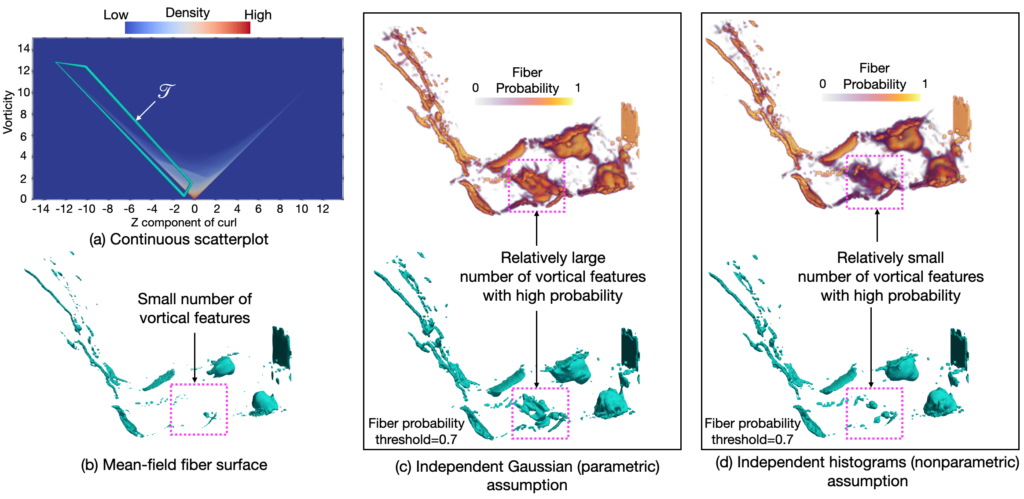Fiber Uncertainty Visualization for Bivariate Data With Parametric and Nonparametric Noise Models
T. M. Athawale, C. R. Johnson, S. Sane, and D. Pugmire
BibTex
(IEEE Transactions on Visualization and Computer Graphics (TVCG), Special Issue on IEEE VIS Conf., vol. 29, no. 1, pp. 613-623, Jan. 2023.)
Abstract

Visualization and analysis of multivariate data and their uncertainty are top research challenges in data visualization. Constructing fiber surfaces is a popular technique for multivariate data visualization that generalizes the idea of level-set visualization for univariate data to multivariate data. In this paper, we present a statistical framework to quantify positional probabilities of fibers extracted from uncertain bivariate fields. Specifically, we extend the state-of-the-art Gaussian models of uncertainty for bivariate data to other parametric distributions (e.g., uniform and Epanechnikov) and more general nonparametric probability distributions (e.g., histograms and kernel density estimation) and derive corresponding spatial probabilities of fibers. In our proposed framework, we leverage Green's theorem for closed-form computation of fiber probabilities when bivariate data are assumed to have independent parametric and nonparametric noise. Additionally, we present a nonparametric approach combined with numerical integration to study the positional probability of fibers when bivariate data are assumed to have correlated noise. For uncertainty analysis, we visualize the derived probability volumes for fibers via volume rendering and extracting level sets based on probability thresholds. We present the utility of our proposed techniques via experiments on synthetic and simulation datasets.Quantifying and Visualizing Uncertainty for Source Localization in Electrocardiographic Imaging
D. Njeru, T. M. Athawale, J. France, and C. R. Johnson

(Accepted for publication in Computer Methods in Biomechanics and Biomedical Engineering: Imaging and Visualization, 2022)
Abstract

Electrocardiographic imaging (ECGI) presents a clinical opportunity to noninvasively understand the sources of arrhythmias for individual patients. To help increase the effectiveness of ECGI, we provide new ways to visualize associated measurement and modeling errors. In this paper, we study source localization uncertainty in two steps: First, we perform Monte Carlo simulations of a simple inverse ECGI source localization model with error sampling to understand the variations in ECGI solutions. Second, we present multiple visualization techniques, including confidence maps, level-sets, and topology-based visualizations, to better understand uncertainty in source localization. Our approach offers a new way to study uncertainty in the ECGI pipeline.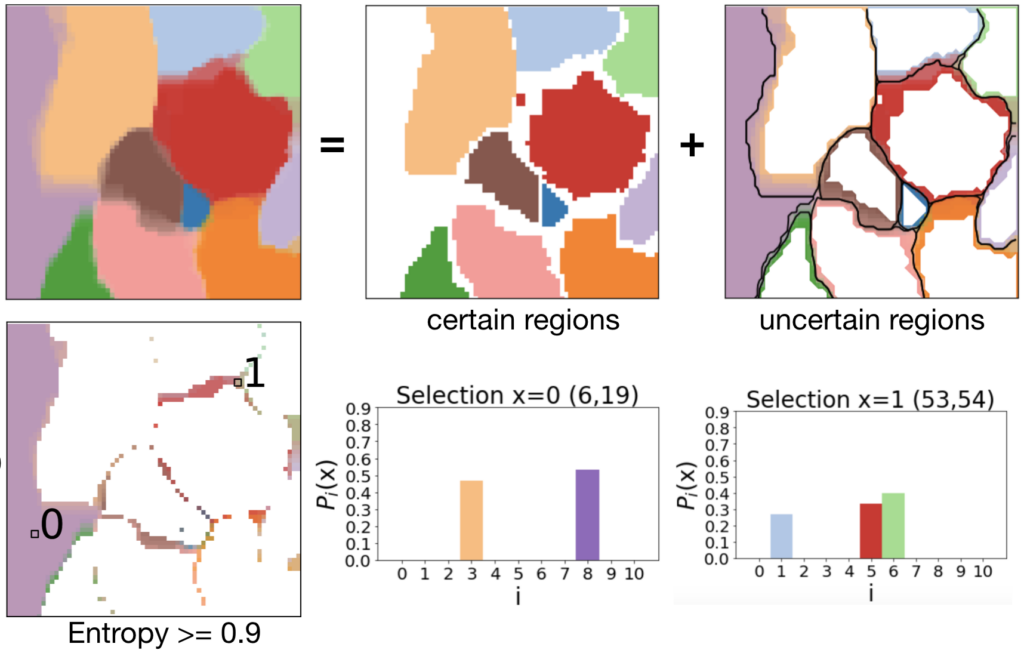Uncertainty Visualization of 2D Morse Complex Ensembles Using Statistical Summary Maps
T. M. Athawale, D. Maljovec, L. Yan, C. R. Johnson, V. Pascucci, and B. Wang
] [bibTex] [Preview video] [PubMed] [Presentation slides] [Presentation video (VIS 2020 uncertainty session, talk# 2)]
(IEEE Transactions on Visualization and Computer Graphics (TVCG), vol. 28, no. 4, pp. 1955-1966, 2022)
Abstract

Morse complexes are gradient-based topological descriptors with close connections to Morse theory. They are widely applicable in scientific visualization as they serve as important abstractions for gaining insights into the topology of scalar fields. Data uncertainty inherent to scalar fields due to randomness in their acquisition and processing, however, limits our understanding of Morse complexes as structural abstractions. We, therefore, explore uncertainty visualization of an ensemble of 2D Morse complexes that arises from scalar fields coupled with data uncertainty. We propose several statistical summary maps as new entities for quantifying structural variations and visualizing positional uncertainties of Morse complexes in ensembles.  Specifically, we introduce three types of statistical summary maps -- the probabilistic map, the significance map, and the survival map -- to characterize the uncertain behaviors of gradient flows. We demonstrate the utility of our proposed approach using wind, flow, and ocean eddy simulation datasets.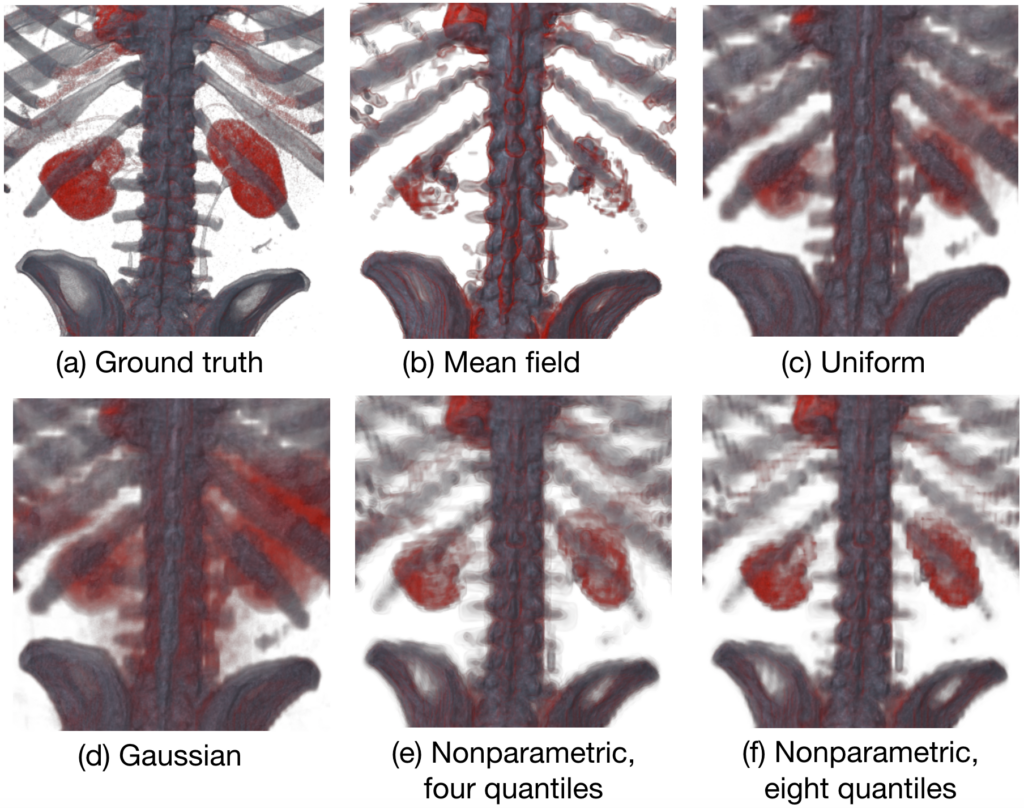Direct Volume Rendering with Nonparametric Models of Uncertainty
T. M. Athawale, B. Ma, E. Sakhaee, C. R. Johnson, and A. Entezari
(VIS 2020 uncertainty session, talk# 1)]
(IEEE Transactions on Visualization and Computer Graphics (TVCG), Special Issue on IEEE VIS Conf., vol. 27, no. 2, pp. 1797-1807, Feb. 2021.)
Abstract

We present a nonparametric statistical framework for the quantification, analysis, and propagation of data uncertainty in direct volume rendering (DVR). The state-of-the-art statistical DVR framework allows for preserving the transfer function (TF) of the ground truth function when visualizing uncertain data; however, the existing framework is restricted to parametric models of uncertainty. In this paper, we address the limitations of the existing DVR framework by extending the DVR framework for nonparametric distributions. We exploit the quantile interpolation technique to derive probability distributions representing uncertainty in viewing-ray sample intensities in closed form, which allows for accurate and efficient computation. We evaluate our proposed nonparametric statistical models through qualitative and quantitative comparisons with the mean-field and parametric statistical models, such as uniform and Gaussian, as well as Gaussian mixtures. In addition, we present an extension of the state-of-the-art rendering parametric framework to 2D TFs for improved DVR classifications. We show the applicability of our uncertainty quantification framework to ensemble, downsampled, and bivariate versions of scalar field datasets.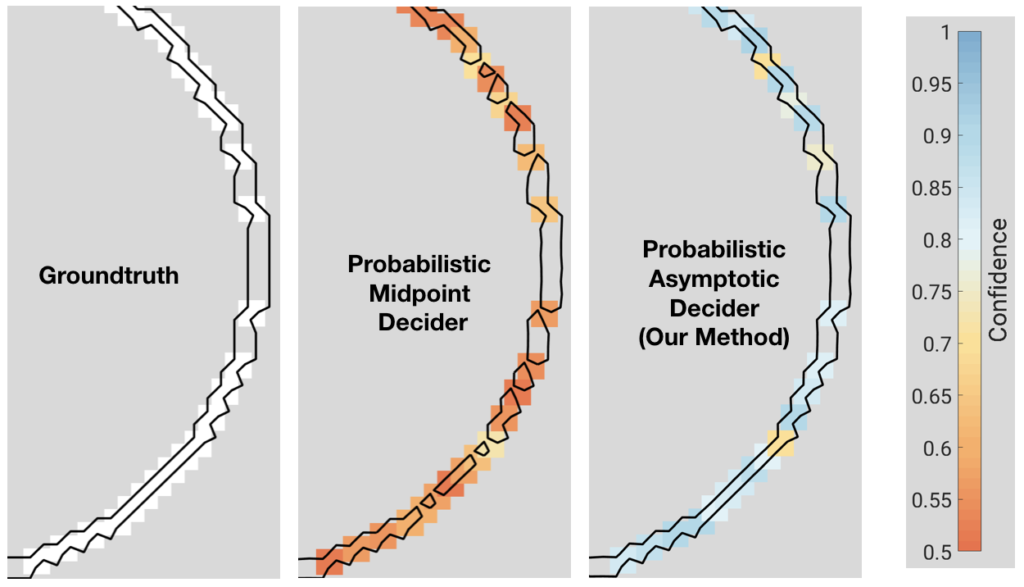Probabilistic Asymptotic Decider for Topological Ambiguity Resolution in Level-Set Extraction for Uncertain 2D Data
T. M. Athawale and C. R. Johnson
BibTex]
(IEEE Transactions on Visualization and Computer Graphics (TVCG), Special Issue on IEEE VIS Conf, vol. 25, no. 1, pp. 1163-1172, Jan. 2019.)
Abstract

We present a framework for the analysis of uncertainty in isocontour extraction. The marching squares (MS) algorithm for isocontour reconstruction generates a linear topology that is consistent with hyperbolic curves of a piecewise bilinear interpolation. The saddle points of the bilinear interpolant cause topological ambiguity in isocontour extraction. The midpoint decider and the asymptotic decider are well-known mathematical techniques for resolving topological ambiguities. The latter technique investigates the data values at the cell saddle points for ambiguity resolution. The uncertainty in data, however, leads to uncertainty in underlying bilinear interpolation functions for the MS algorithm, and hence, their saddle points. In our work, we study the behavior of the asymptotic decider when data at grid vertices is uncertain. First, we derive closed-form distributions characterizing variations in the saddle point values for uncertain bilinear interpolants. The derivation assumes uniform and nonparametric noise models, and it exploits the concept of ratio distribution for analytic formulations. Next, the probabilistic asymptotic decider is devised for ambiguity resolution in uncertain data using distributions of the saddle point values derived in the first step. Finally, the confidence in probabilistic topological decisions is visualized using a colormapping technique. We demonstrate the higher accuracy and stability of the probabilistic asymptotic decider in uncertain data with regard to existing decision frameworks, such as deciders in the mean field and the probabilistic midpoint decider, through the isocontour visualization of synthetic and real datasets.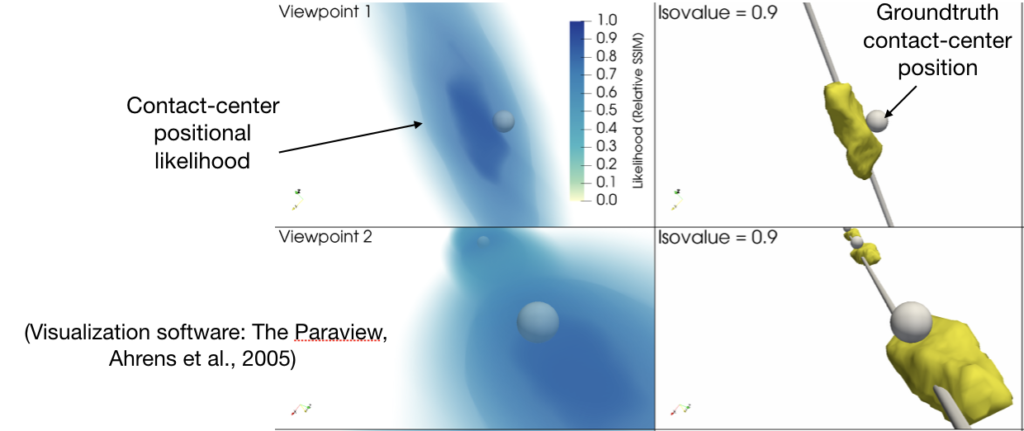A Statistical Framework for Quantification and Visualization of Positional Uncertainty in Deep Brain Stimulation Electrodes
T. M. Athawale, K. A. Johnson, C. R. Butson, and C. R. Johnson
[Preprint] [BibTex] [PubMed] [Poster] [Source code (MATLAB)]
(Computer Methods in Biomechanics and Biomedical Engineering: Imaging & Visualization, vol. 7, no. 4, pp. 438-449, 2019.)
Abstract

Deep brain stimulation (DBS) is an established therapy for treating patients with movement disorders such as Parkinson’s disease. Patient-specific computational modeling and visualization have been shown to play a key role in surgical and therapeutic decisions for DBS. The computational models use brain imaging, such as magnetic resonance (MR) and computed tomography (CT), to determine the DBS electrode positions within the patient’s head. The finite resolution of brain imaging, however, introduces uncertainty in electrode positions. The DBS stimulation settings for optimal patient response are sensitive to the relative positioning of DBS electrodes to a specific neural substrate (white/grey matter). In our contribution, we study positional uncertainty in the DBS electrodes for imaging with finite resolution. In a three-step approach, we first derive a closed-form mathematical model characterizing the geometry of the DBS electrodes. Second, we devise a statistical framework for quantifying the uncertainty in the positional attributes of the DBS electrodes, namely the direction of longitudinal axis and the contact-centre positions at subvoxel levels. The statistical framework leverages the analytical model derived in step one and a Bayesian probabilistic model for uncertainty quantification. Finally, the uncertainty in contact-centre positions is interactively visualized through volume rendering and isosurfacing techniques. We demonstrate the efficacy of our contribution through experiments on synthetic and real datasets. We show that the spatial variations in true electrode positions are significant for finite resolution imaging, and interactive visualization can be instrumental in exploring probabilistic positional variations in the DBS lead.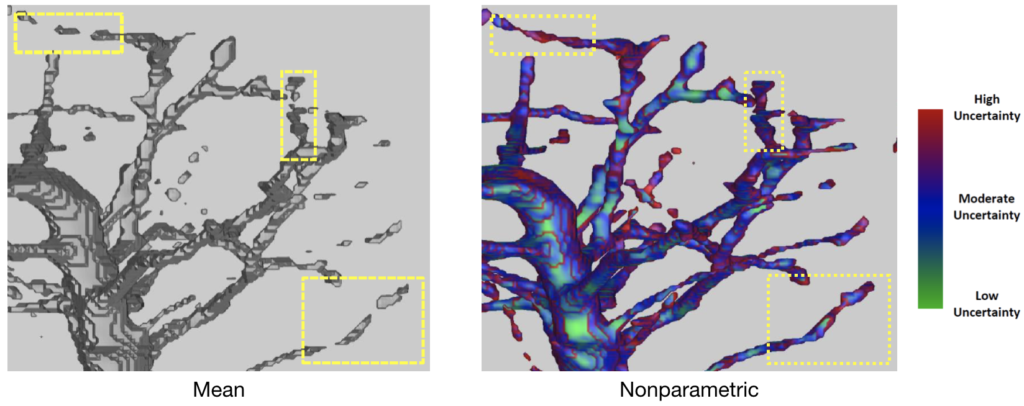Isosurface Visualization of Data with Nonparametric Models for Uncertainty
T. M. Athawale, E. Sakhaee, and A. Entezari

(IEEE Transactions on Visualization and Computer Graphics (TVCG), Special Issue on IEEE VIS Conf, vol. 22, no. 1, pp. 777-786, Jan. 2016.)
Abstract

The problem of isosurface extraction in uncertain data is an important research problem and may be approached in two ways. One can extract statistics (e.g., mean) from uncertain data points and visualize the extracted field. Alternatively, data uncertainty, characterized by probability distributions, can be propagated through the isosurface extraction process. We analyze the impact of data uncertainty on topology and geometry extraction algorithms. A novel, edge-crossing probability based approach is proposed to predict underlying isosurface topology for uncertain data. We derive a probabilistic version of the midpoint decider that resolves ambiguities that arise in identifying topological configurations. Moreover, the probability density function characterizing positional uncertainty in isosurfaces is derived analytically for a broad class of nonparametric distributions. This analytic characterization can be used for efficient closed-form computation of the expected value and variation in geometry. Our experiments show the computational advantages of our analytic approach over Monte-Carlo sampling for characterizing positional uncertainty. We also show the advantage of modeling underlying error densities in a nonparametric statistical framework as opposed to a parametric statistical framework through our experiments on ensemble datasets and uncertain scalar fields.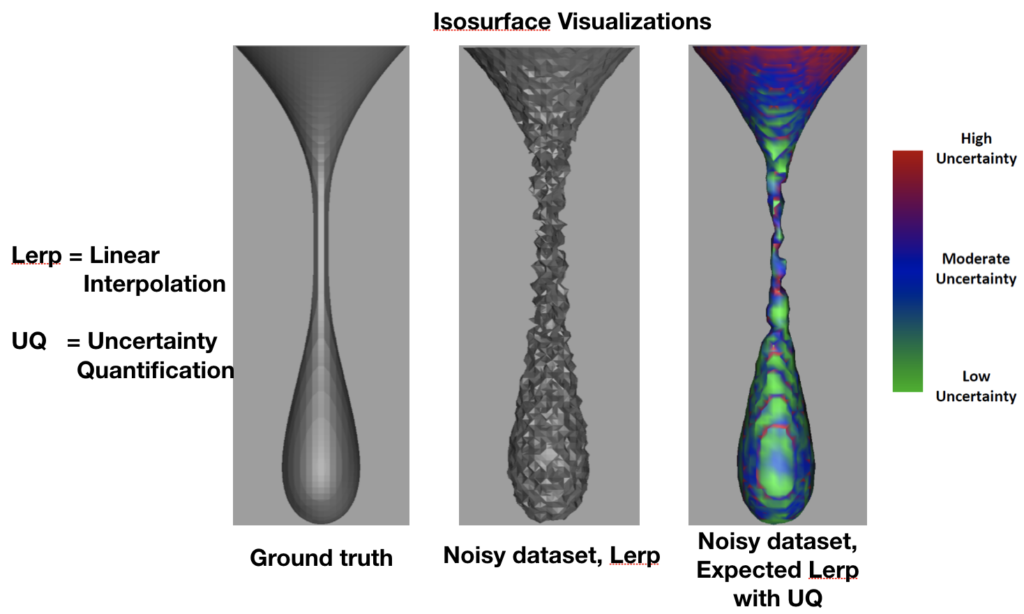Uncertainty Quantification in Linear Interpolation for Isosurface Extraction
T. M. Athawale and A. Entezari

(IEEE Transactions on Visualization and Computer Graphics (TVCG), Special Issue on IEEE VIS Conf, vol. 19, no. 12, pp. 2723-2732, Dec. 2013.)
Abstract

We present a study of linear interpolation when applied to uncertain data. Linear interpolation is a key step for isosurface extraction algorithms, and the uncertainties in the data lead to non-linear variations in the geometry of the extracted isosurface. We present an approach for deriving the probability density function of a random variable modeling the positional uncertainty in the isosurface extraction. When the uncertainty is quantified by a uniform distribution, our approach provides a closed-form characterization of the mentioned random variable. This allows us to derive, in closed form, the expected value as well as the variance of the level-crossing position. While the former quantity is used for constructing a stable isosurface for uncertain data, the latter is used for visualizing the positional uncertainties in the expected isosurface level crossings on the underlying grid.